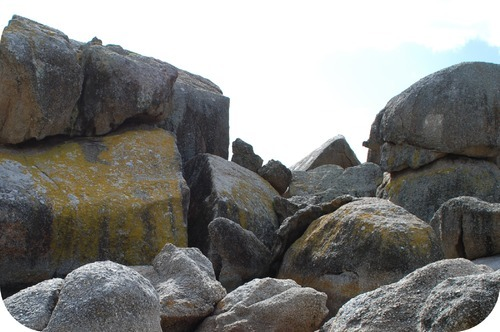## Calculating Atomic Mass

### Learning Objectives

• Define atomic mass.
• Calculate atomic mass given relevant information about the isotopes.

### Have you ever tried to move a boulder?

You have a pile of rocks to move and need to decide what equipment you want to rent to move them.  If the rocks are fairly small, you can get a shovel to pick them up. Larger rocks could be moved by hand, but big boulders will need some sort of mechanical scoop. The amount of each kind of rock will also determine how much time you will need to get the job done. Knowing the relative amounts of large, medium, and small rocks can be very useful in deciding how to approach the job.Most elements occur naturally as a mixture of two or more isotopes. Table below shows the natural isotopes of several elements, along with the percent natural abundance of each.

 Element Isotope (symbol) Percent natural abundance Atomic mass (amu) Average atomic mass (amu) Hydrogen $^1_1\text{H}$ 99.985 1.0078 1.0079 $^2_1\text{H}$ 0.015 2.0141 $^3_1\text{H}$ negligible 3.0160 Carbon $^{12}_6\text{C}$ 98.89 12.000 12.011 $^{13}_6\text{C}$ 1.11 13.003 $^{14}_6\text{C}$ trace 14.003 Oxygen $^{16}_8\text{O}$ 99.759 15.995 15.999 $^{17}_8\text{O}$ 0.037 16.995 $^{18}_8\text{O}$ 0.204 17.999 Chlorine $^{35}_{17}\text{Cl}$ 75.77 34.969 35.453 $^{37}_{17}\text{Cl}$ 24.23 36.966 Copper $^{63}_{29}\text{Cu}$ 69.17 62.930 63.546 $^{65}_{29}\text{Cu}$ 30.83 64.928

For some elements, one particular isotope predominates greatly over the other isotopes. Naturally occurring hydrogen is nearly all hydrogen-1 and naturally occurring oxygen is nearly all oxygen-16. For many other elements, however, more than one isotope may exist in more substantial quantities. Chlorine (atomic number 17) is a yellowish-green toxic gas. About three quarters of all chlorine atoms have 18 neutrons, giving those atoms a mass number of 35. About one quarter of all chlorine atoms have 20 neutrons, giving those atoms a mass number of 37. Were you to simply calculate the arithmetic average of the precise atomic masses, you would get 36.

$\displaystyle\frac{\left(34.969+36.966\right)}{2}=35.968\text{ amu}$

Clearly the actual average atomic mass from the last column of the table is significantly lower. Why? We need to take into account the percent natural abundances of each isotope in order to calculate what is called the weighted average. The atomic mass of an element is the weighted average of the atomic masses of the naturally occurring isotopes of that element. The sample problem below demonstrates how to calculate the atomic mass of chlorine.

Sample Problem: Calculating Atomic Mass

Use the atomic masses of each of the two isotopes of chlorine along with their percent abundances to calculate the average atomic mass of chlorine.

Step 1: List the known and unknown quantities and plan the problem.

Known

• chlorine-35: atomic mass = 34.969 amu and % abundance = 75.77%
• chlorine-37: atomic mass = 36.966 amu and % abundance = 24.23%

Unknown

• Average atomic mass of chlorine

Change each percent abundance into decimal form by dividing by 100. Multiply this value by the atomic mass of that isotope. Add together for each isotope to get the average atomic mass.

Step 2: Calculate

 chlorine-35 0.7577 × 34.969 = 26.50 amu chlorine-37 0.2423 × 26.966 = 8.957 amu average atomic mass 26.50 + 8.957 = 35.45 amu

Note: Applying significant figure rules results in the 35.45 amu result without excessive rounding error. In one step:

(0.7577 × 34.969) + (0.2423 × 36.966) = 35.45 amu

The calculated average atomic mass is closer to 35 than to 37 because a greater percentage of naturally occurring chlorine atoms have the mass number of 35. It agrees with the value from the Table above .

### Summary

• The atomic mass of an element is the weighted average of the atomic masses of the naturally occurring isotopes of that element.
• Calculations of atomic mass use the percent abundance of each isotope.

### Practice

Click on the link below to get some experience in atomic mass determinations: